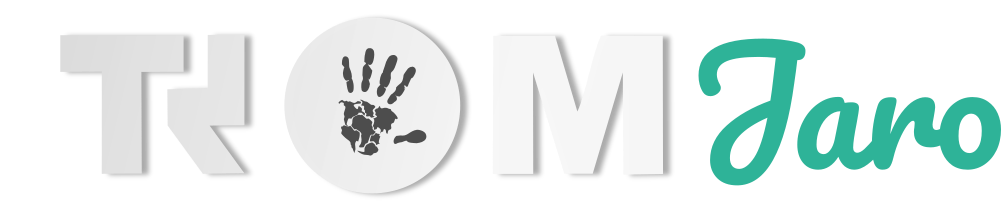## Plots

Plots is a graph plotting app for GNOME. Plots makes it easy to visualise mathematical formulae.

## Xaos

XaoS (pronounced chaos) lets you dive into fractals in one fluid, continuous motion.

## Step

Step is an interactive physical simulator. It allows you to explore the physical world through simulations.

## Kig

Kig is an interactive mathematics software for learning and teaching geometry.

## Qalculate

Qalculate! is a multi-purpose cross-platform desktop calculator. It is simple to use but provides power and versatility normally reserved for complicated math packages, as well as useful tools for everyday needs (such as currency conversion and percent calculation).

## GeoGebra

Get our free online math tools for graphing, geometry, 3D, and more!